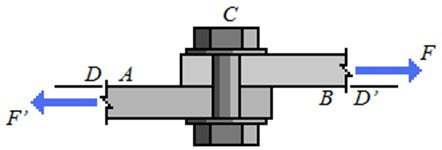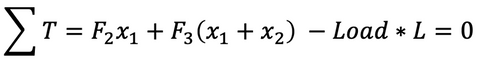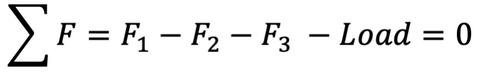# How Much Weight Can Drawer Slides Hold?

In any project where you plan to use drawer slides to provide telescopic motion, you’ll inevitably ask yourself how much weight can these drawer slides hold? Or will these drawer slides hold my desired weight? This blog will aim to help answer those questions and help you ensure your next drawer slide project goes smoothly. If you need to learn more about the basics of drawer slides, check out our Drawer Slides 101 blog.

When you go to purchase drawer slides for your project, your selected drawer slides will come with a load rating or load capacity specification. This specification is the maximum allowable load or weight the drawer slide can handle before failure. These values will depend on the type of drawer slide you choose, but if you’re worried about how much weight your drawer slides can handle it is best to go with ball-bearing drawer slides as they have much higher load ratings. You can also find heavy duty versions of ball-bearing drawer slides which provide even greater load ratings compared to regular drawer slides.## Determining How Much Do You Need Your Drawer Slides to Carry?

Determining the amount of weight that your drawer slide will carry is key in selecting the right drawer slide for your application. If you are side mounting your pair of drawer slides, like in a typical cabinet, the calculation to determine how much weight each drawer slide is carrying is pretty straight forward. The free body diagram below shows a typical cabinet carrying a 500lbs load with a drawer slide on either side supporting the cabinet. Balancing the forces that are applied to the cabinet, the force exerted by one drawer slide (F) is equal to half of the weight or 250lbs. This means a drawer slide with a load rating greater than 250lbs will work within our application.### Bottom Mounting

If you want to have your drawer slides mounted to the bottom of the cabinet, you can do so with some ball-bearing drawer slides. Although, this will impact the load rating that those drawer slides can carry due to the change in orientation. With side mounting, the compression force from the weight being carried by drawer slides is transferred from each member through the ball-bearings. While with bottom mounting, this compression force is supported solely by the structure of the drawer slides. Because of this, the drawer slides are stronger in the vertical configuration, which utilizes the ball-bearings, compared to the horizontal configuration. Which means when in the horizontal configuration, like for bottom mounting, the drawer slides will have a smaller load rating compared to the vertical configuration.While your selected drawer slide might be able to hold 250lbs, this doesn’t mean your design will. The load carried by your drawer slides will cause a shear stress on the mounting fasteners for your drawer slides. If the shear stress on your mounting fasteners is greater than that fastener’s shear yield strength, the fasteners will fail. Shear yield strength is the amount of shear stress the fastener can handle before failure and is based on material properties of the fastener. Shear stress is equal to the shearing force times the cross-sectional area of the fastener. The shearing force is the reactionary force of the fastener to support the drawer slide.Below is the free body diagram for a fully extended drawer slide that is fastened to a cabinet with 3 fasteners. As we discussed above, the load on one drawer slide is half of the weight that the pair of slides are carrying. To determine each force on each of the fasteners, we’ll need to use both the balance of forces and torques equations. In balance of torques equation, the point of rotation would be about the first fastener, so that force (F1) will not apply a torque. As the first fastener is the point of rotation, the forces from the other two fasteners will act downwards to counteract the torque caused by the load and keep the drawer slide level. Now balancing the forces, we can determine that the force from the first fastener will be equal to the sum of the forces from the other two fasteners and the load.Balance of Torques:Balance of Forces:Unfortunately, this is an indeterminate problem as there are 3 unknowns and only two equations to relate them. If you only used 2 fasteners to mount your drawer slides, which is not recommended, you’ll be able to solve this problem. You can estimate the forces either by trial and error, using techniques to solve indeterminate beam problems, or by simplifying the problem down to just two fasteners. Either way, once you have an estimate of the force on each fastener you can calculate the shear stress and check to ensure it isn’t larger than the shear yield strength of the fastener. Calculating the shear stress on each fastener is particularly important for heavy loads and may not be necessary for lighter applications.

 Image from: http://www.engineeringarchives.com/les_mom_singledoubleshear.html